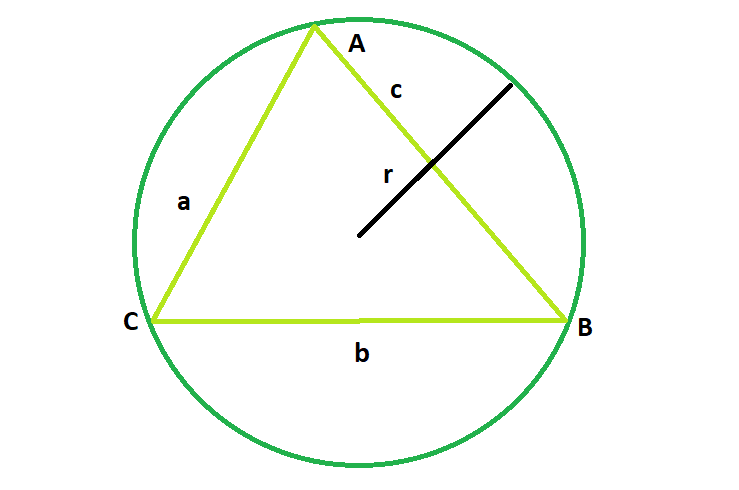# Area of the circumcircle of any triangles with sides given

Given a triangle with known sides a, b and c; the task is to find the area of its circumcircle.

Examples:

Input: a = 2, b = 2, c = 3
Output: 7.17714

Input: a = 4, b = 5, c = 3
Output: 19.625Approach:
For a triangle with side lengths a, b, and c,

Radius of the circumcircle:where A = √(s*(s-a)*(s-b)*(s-c))
and s = (a+b+c)/2 is the semiperimeter.

Therefore, Area of the circumcircle:Below is the implementation of the above approach:

## C++

 // C++ Program to find the area  // the circumcircle of the given triangle     #include  using namespace std;     // Function to find the area  // of the circumcircle  float circlearea(float a, float b, float c)  {         // the sides cannot be negative      if (a < 0 || b < 0 || c < 0)          return -1;         // semi-perimeter of the circle      float p = (a + b + c) / 2;         // area of triangle      float At = sqrt(p * (p - a) * (p - b) * (p - c));         // area of the circle      float A = 3.14 * pow(((a * b * c) / (4 * At)), 2);      return A;  }     // Driver code  int main()  {         // Get the sides of the triangle      float a = 4, b = 5, c = 3;         // Find and print the area of the circumcircle      cout << circlearea(a, b, c) << endl;         return 0;  }

## Java

 // Java Program to find the area  // the circumcircle of the given triangle  import java.*;  class gfg  {  // Function to find the area  // of the circumcircle  public double circlearea(double a, double b, double c)  {         // the sides cannot be negative      if (a < 0 || b < 0 || c < 0)          return -1;         // semi-perimeter of the circle      double p = (a + b + c) / 2;         // area of triangle      double At = Math.sqrt(p * (p - a) * (p - b) * (p - c));         // area of the circle      double A = 3.14 * Math.pow(((a * b * c) / (4 * At)), 2);      return A;  }  }     class geek  {  // Driver code  public static void main(String[] args)  {      gfg g = new gfg();      // Get the sides of the triangle      double a = 4, b = 5, c = 3;         // Find and print the area of the circumcircle      System.out.println(g.circlearea(a, b, c));     }  }     //This code is contributed by shk..

## Python3

 # Python3 Program to find the area  # the circumcircle of the given triangle  import math     # Function to find the area  # of the circumcircle  def circlearea(a, b, c):         # the sides cannot be negative      if (a < 0 or b < 0 or c < 0):          return -1;         # semi-perimeter of the circle      p = (a + b + c) / 2;         # area of triangle      At = math.sqrt(p * (p - a) *                    (p - b) * (p - c));         # area of the circle      A = 3.14 * pow(((a * b * c) / (4 * At)), 2);      return A;     # Driver code     # Get the sides of the triangle  a = 4;  b = 5;  c = 3;     # Find and print the area   # of the circumcircle  print (float(circlearea(a, b, c)));     # This code is contributed   # by Shivi_Aggarwal

## C#

 // C# Program to find the area  // the circumcircle of the given triangle  using System;  class gfg  {   // Function to find the area   // of the circumcircle   public double circlearea(double a, double b, double c)   {         // the sides cannot be negative      if (a < 0 || b < 0 || c < 0)          return -1;         // semi-perimeter of the circle      double p = (a + b + c) / 2;         // area of triangle      double At = Math.Sqrt(p * (p - a) * (p - b) * (p - c));         // area of the circle      double A = 3.14 * Math.Pow(((a * b * c) / (4 * At)), 2);      return A;   }  }     class geek  {   // Driver code   public static int Main()   {      gfg g = new gfg();      // Get the sides of the triangle      double a = 4, b = 5, c = 3;         // Find and print the area of the circumcircle      Console.WriteLine(g.circlearea(a, b, c));         return 0;   }  }  //This code os contributed by SoumikMondal

## PHP

 

Output:

19.625


Attention reader! Don’t stop learning now. Get hold of all the important DSA concepts with the DSA Self Paced Course at a student-friendly price and become industry ready.

My Personal Notes arrow_drop_upProgram Analyst Trainee,Cognizant

If you like GeeksforGeeks and would like to contribute, you can also write an article using contribute.geeksforgeeks.org or mail your article to contribute@geeksforgeeks.org. See your article appearing on the GeeksforGeeks main page and help other Geeks.

Please Improve this article if you find anything incorrect by clicking on the "Improve Article" button below.

Article Tags :
Practice Tags :

Be the First to upvote.

Please write to us at contribute@geeksforgeeks.org to report any issue with the above content.